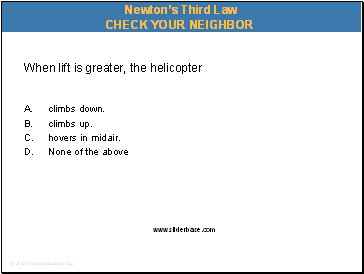# Newton’s laws of motionPage 8

#### WATCH ALL SLIDES

Two people of equal mass on slippery ice push off from each other. Will both move at the same speed in opposite directions?

A. Yes.

Yes, but only if both push equally.

No.

No, unless acceleration occurs.

Explanation:

However they push, the result is equal-magnitude forces on equal masses, which produce equal accelerations and, therefore, equal changes in speed.

Newton’s Third Law of Motion

Slide 61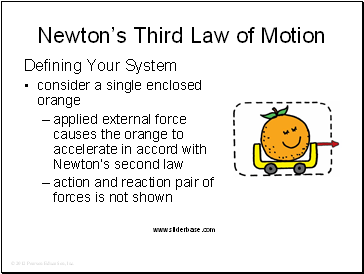Newton’s Third Law of Motion

consider a single enclosed orange

applied external force causes the orange to accelerate in accord with Newton’s second law

action and reaction pair of forces is not shown

Slide 62Newton’s Third Law of Motion

consider the orange and the apple pulling on it

action and reaction do not cancel (because they act on different things)

external force by apple accelerates the orange

Slide 63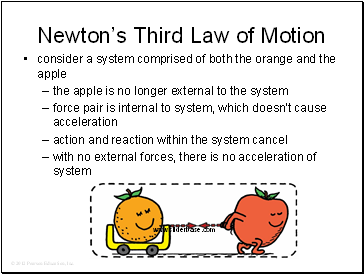Newton’s Third Law of Motion

consider a system comprised of both the orange and the apple

the apple is no longer external to the system

force pair is internal to system, which doesn’t cause acceleration

action and reaction within the system cancel

with no external forces, there is no acceleration of system

Slide 64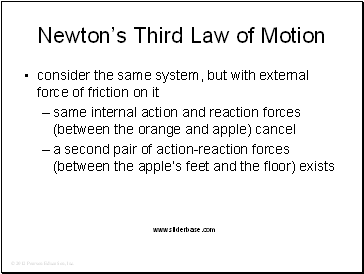Newton’s Third Law of Motion

consider the same system, but with external force of friction on it

same internal action and reaction forces (between the orange and apple) cancel

a second pair of action-reaction forces (between the apple’s feet and the floor) exists

Slide 65Newton’s Third Law of Motion

one of these acts by the system (apple on the floor) and the other acts on the system (floor on the apple)

external frictional force of floor pushes on the system, which accelerates

second pair of action and reaction forces do not cancel

Slide 66When lift equals the weight of a helicopter, the helicopter

A. climbs down.

climbs up.

hovers in midair.

None of the above.

Newton’s Third Law

Slide 67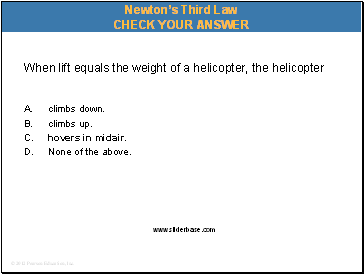When lift equals the weight of a helicopter, the helicopter

A. climbs down.

climbs up.

hovers in midair.

None of the above.

Newton’s Third Law